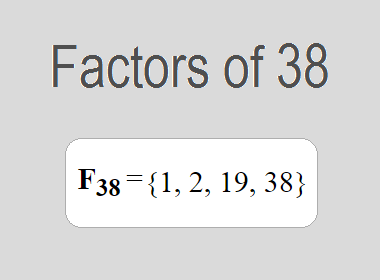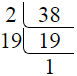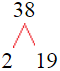# Factors of 38The factors of 38 are 1, 2, 19, and 38 i.e. F38 = {1, 2, 19, 38}. The factors of 38 are all the numbers that can divide 38 without leaving a remainder.

We can check if these numbers are factors of 38 by dividing 38 by each of them. If the result is a whole number, then the number is a factor of 38. Let's do this for each of the numbers listed above:

·        1 is a factor of 38 because 38 divided by 1 is 38.

·        2 is a factor of 38 because 38 divided by 2 is 19.

·        19 is a factor of 38 because 38 divided by 19 is 2.

·        38 is a factor of 38 because 38 divided by 38 is 1.

## How to Find Factors of 38?

1 and the number itself are the factors of every number. So, 1 and 38 are two factors of 38. To find the other factors of 38, we can start by dividing 38 by the numbers between 1 and 38. If we divide 38 by 2, we get a remainder of 0. Therefore, 2 is a factor of 38. If we divide 38 by 3, we get a remainder of 2. Therefore, 3 is not a factor of 38.

Next, we can check if 4 is a factor of 38. If we divide 38 by 4, we get a remainder of 2. Therefore, 4 is not a factor of 38. We can continue this process for all the possible factors of 38.

Through this process, we can find that the factors of 38 are 1, 2, 19, and 38. These are the only numbers that can divide 38 without leaving a remainder.

********************

********************

## Properties of the Factors of 38

The factors of 38 have some interesting properties. One of the properties is that the sum of the factors of 38 is equal to 60. We can see this by adding all the factors of 38 together:

1 + 2 + 19 + 38 = 60

Another property of the factors of 38 is that the prime factors of 38 are 2 and 19.

## Applications of the Factors of 38

The factors of 38 have several applications in mathematics. One of the applications is in finding the highest common factor (HCF) of two or more numbers. The HCF is the largest factor that two or more numbers have in common. For example, to find the HCF of 38 and 19, we need to find the factors of both numbers and identify the largest factor they have in common. The factors of 38 are 1, 2, 19, and 38. The factors of 19 are 1, and 19. The largest factor that they have in common is 19. Therefore, the HCF of 38 and 19 is 19.

Another application of the factors of 38 is in prime factorization. Prime factorization is the process of expressing a number as the product of its prime factors. The prime factors of 38 are 2 and 19, since these are the only prime numbers that can divide 38 without leaving a remainder. Therefore, we can express 38 as:

38 = 2 × 19

We can do prime factorization by division and factor tree method also. Here is the prime factorization of 38 by division method,38 = 2 × 19

Here is the prime factorization of 38 by the factor tree method,38 = 2 × 17

## Conclusion

The factors of 38 are the numbers that can divide 38 without leaving a remainder. The factors of 38 are 1, 2, 19, and 38. The factors of 38 have some interesting properties, such as having a sum of 60. The factors of 38 have several applications in mathematics, such as finding the highest common factor and prime factorization.